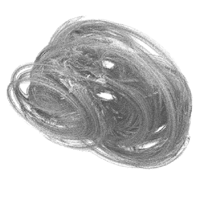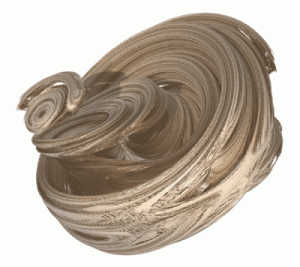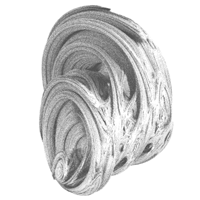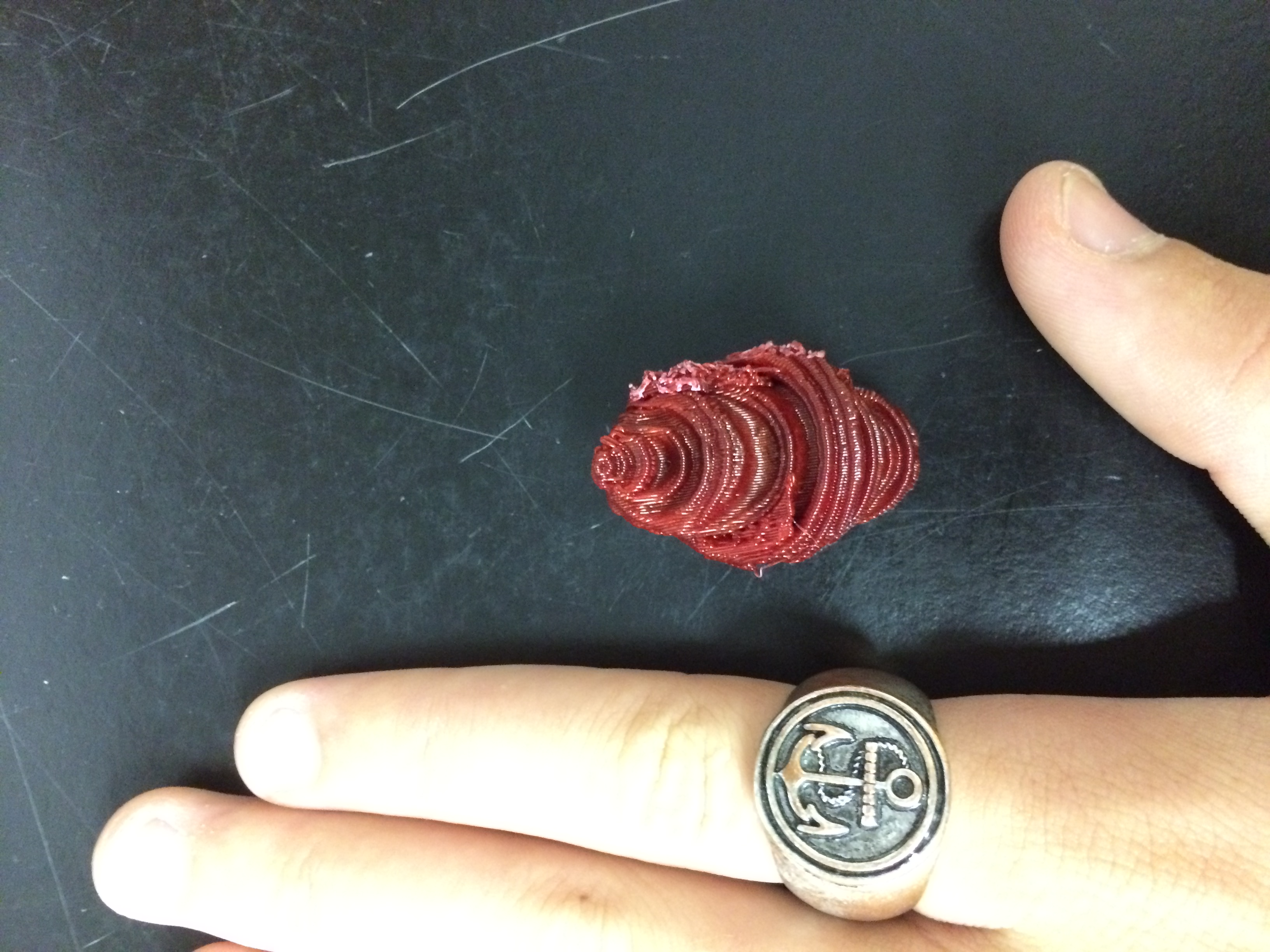# Quaternion Julia

The first fractal I want to print is the Quaternion Julia fractal. According to an website written by Paul Bourke: “A quaternion has two more complex components and might be written as q = r + a i + b j + c k where r, a, b, and c are real numbers. There are slightly more complicated relationships between i, j, and kA quaternion has two more complex components and might be written as q = r + a i + b j + c k where r, a, b, and c are real numbers. There are slightly more complicated relationships between i, j, and k

i2 = j2 = k2 = -1

i j = k     j k = i

k i = j     j i = -k

k j = -i      i k = -j.

To generate a quaternion fractal a function is iterated zn+1 = f(zn) and if it tends to infinity then it is outside the Julia set, if it is bounded then it is inside the set.” I personally think this is a bit complicated, as I have forgotten a lot about complex and imaginary numbers but the concept regarding how it interacts with limits still makes sense to me.After watching a few videos and reading up on the calculus behind this complex, but beautiful, fractal I found this video that looped the different stages of a Quaternion Julia fractal.

https://youtu.be/VkmqT6MQoDE

This model was created by theswope on Thingiverse with a very vague description not disclosing how he made it. It doesnt say how big the model is but i believe it is small, as it is very polygonal and he has other older versions that are not as printer friendly he called “tests”.When I printed this fractal, I had to shrink it down a lot and I printed it on the Afinia which added a ton of supports. I want to try and scale it up and printing it on the Ultimaker eventually to get a more accurate print. My hand is visible for reference. Here is my make on Thingiverse.